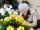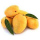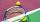# Ornamental shrubs

Children committed to planting 240 ornamental shrubs. Their commitment, however, exceeded by 48 shrubs. Write the ratio of actually planted shrubs and commitment by lowest possible integers a/b.

a =  6
b =  5

### Step-by-step explanation:

$b=x\mathrm{/}d=240\mathrm{/}48=5$Did you find an error or inaccuracy? Feel free to write us. Thank you!Tips to related online calculators
Do you want to calculate greatest common divisor two or more numbers?
Need help to calculate sum, simplify or multiply fractions? Try our fraction calculator.
Check out our ratio calculator.
Do you want to perform natural numbers division - find the quotient and remainder?

## Related math problems and questions:

• Sports studentsThere are 120 athletes, 48 volleyball players, and 72 handball players at the school with extended sports training. Is it possible to divide sports students into groups so that the number in each group is the same and expressed by the largest possible num
• PegsFrom two sticks 240 cm and 210 cm long, it is necessary to cut the longest possible pegs for flowers so that no residues remain. How many pegs will it be?
• On Children'sOn Children's Day, the organizers bought 252 chewing gums, 396 candies and 108 lollipops. They want to make as many of the same packages as possible. Advise them what to put in each package and how many packages they can make this way.
• FloristFlorist has 84 red and 48 white roses. How many same bouquets can he make from them if he must use all roses?
• Common divisorsFind all common divisors of numbers 30 and 45.The local reader’s club has a set of 28 hardback books and a set of 44 paperbacks. Each set can be divided equally among the club members. What is the greatest possible number of club members?
• 6/24 change6/24 change to a fraction in lowest terms
• Paper squaresThe paper rectangle measuring 69 cm and 46 cm should be cut into as many squares as possible. Calculate the lengths of squares and their number.
• Divisible by fourAre 240 divisible by 4?
• LCD 2The least common denominator of 2/5, 1/2, and 3/4
• BuildingAt the building, we divided 240 boards into two piles in a 5: 3 ratio. How many were fewer boards in the lower pile?
• Ratio6 numbers are in the ratio 1:5:1:5:5:5. Their sum is 242. What are the numbers?
• School booksAt the beginning of the school year, the teacher distributed 480 workbooks and 220 textbooks. How many pupils could have the most in the classroom?
• MangoesChris has 12 mangoes and Jay has 18 mangoes. Each of them will share the mangoes to their friends . What's the greatest number of mangoes each of their friends get if Chris and Jay will give the same number of mangoes?
• How manyHow many integers are greater than 547/3 and less than 931/4?The children of the tennis school received 64 white and 48 yellow balls from the sponsor. When asked about how many balls they could take, they were answered: "You have so many that none of you will have more than 10 balls and all will have the same numbeThree friends had balls in ratio 2: 7: 4 at the start of the game. Could they have the same number of balls at the end of the game? Write 0, if not, or write the minimum number of balls they had together.